# Solution assignment 10 Area and volume

### Assignment 10

Suppose we have a sphere with radius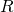. We drill a cylinder in this sphere. The axis of the cylinder goes through the center of the sphere (compare a corer). After the drill it appears that the cylinder has a height ofwith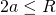. Calculate the volume of the spherical shell that remains after the cylinder has been drilled.

### Solution

We assume that the center of the sphere is the origin. Then we look at the first quadrant and see that the cylinder goes from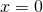to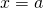. The radius of the cylinder is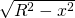. The volume of the spherical shell is then: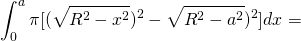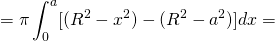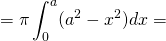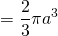And thus the content of the spherical shell is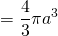Note that the this result is independent of the radius of the sphere. We can verify that this result may be correct: if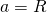then the cylinder is infinitely thin and then the volume of the cylinder equals the volume of the sphere.  If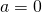then the height of the cylinder is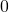and thus we drilled the complete sphere and the remaining spherical shell equals.